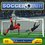Collinearity

Let $a_{1}, a_{2}, a_{3},....a_{n}$ and $b_{1} , b_{2}, b_{3},...b_{n}$ be two arithmetic progressions.

Then prove that the points : $(a_{1},b_{1} ),(a_{2},b_{2}),.......,(a_{n},b_{n})$ are collinear.Note by Aditya Parson
6 years, 5 months ago

This discussion board is a place to discuss our Daily Challenges and the math and science related to those challenges. Explanations are more than just a solution — they should explain the steps and thinking strategies that you used to obtain the solution. Comments should further the discussion of math and science.

When posting on Brilliant:

• Use the emojis to react to an explanation, whether you're congratulating a job well done , or just really confused .
• Ask specific questions about the challenge or the steps in somebody's explanation. Well-posed questions can add a lot to the discussion, but posting "I don't understand!" doesn't help anyone.
• Try to contribute something new to the discussion, whether it is an extension, generalization or other idea related to the challenge.
• Stay on topic — we're all here to learn more about math and science, not to hear about your favorite get-rich-quick scheme or current world events.

MarkdownAppears as
*italics* or _italics_ italics
**bold** or __bold__ bold

- bulleted
- list

• bulleted
• list

1. numbered
2. list

1. numbered
2. list
Note: you must add a full line of space before and after lists for them to show up correctly
paragraph 1

paragraph 2

paragraph 1

paragraph 2

> This is a quote
This is a quote
# I indented these lines
# 4 spaces, and now they show
# up as a code block.

print "hello world"
# I indented these lines
# 4 spaces, and now they show
# up as a code block.

print "hello world"
MathAppears as
Remember to wrap math in $$...$$ or $...$ to ensure proper formatting.
2 \times 3 $2 \times 3$
2^{34} $2^{34}$
a_{i-1} $a_{i-1}$
\frac{2}{3} $\frac{2}{3}$
\sqrt{2} $\sqrt{2}$
\sum_{i=1}^3 $\sum_{i=1}^3$
\sin \theta $\sin \theta$
\boxed{123} $\boxed{123}$

Sort by:

We will now show that any 3 points are collinear.

Thus, we have to show $(a_k, b_k)$, $(a_m, b_m)$ and $(a_n, b_n)$ are collinear.

$\iff$ Line formed by $(a_k, b_k)$ and $(a_m, b_m)$ is parallel to line formed by $(a_m, b_m)$ and $(a_n, b_n)$

Using the fact of arithmetic progressions, we can let $a_k=a_0+k \times d_a$ and $b_k=b_0+k \times d_b$, for some constants $a_0, d_a, b_0, d_b$

Therefore we can show that the gradients of the 2 lines are equal.

Gradient of line formed by $(a_k, b_k)$ and $(a_m, b_m)=\frac{b_m-b_k}{a_m-a_k}=\frac{d_b}{d_a}$

Gradient of line formed by $(a_m, b_m)$ and $(a_n, b_n)=\frac{b_m-b_n}{a_m-a_n}=\frac{d_b}{d_a}$

Since gradients are equal, it follows that the lines are parallel and thus $(a_k, b_k)$, $(a_m, b_m)$ and $(a_n, b_n)$ are collinear.

Now we can start with base case n=3 and induct by taking points $(a_1, b_1), (a_2, b_2)$ and $(a_{n+1}, b_{n+1})$. Hence proven.

- 6 years, 5 months ago

I will corroborate your solution there.

- 6 years, 5 months ago

Let $a_i = \lambda_a i+\mu_a$, $b_i = \lambda_b i+\mu_b$ for some real numbers $\lambda_a,\lambda_b,\mu_a,\mu_b$.

Then $i=\frac{a_i-\mu_a}{\lambda_a}$, and thus $b_i = \lambda_b\frac{a_i-\mu_a}{\lambda_a}+\mu_b = \frac{\lambda_b}{\lambda_a}a_i+(\mu_b-\frac{\mu_a\lambda_b}{\lambda_a})$.

Hence all the points $(a_i,b_i)$ lie on the same line $y=\frac{\lambda_b}{\lambda_a}x+(\mu_b-\frac{\mu_a\lambda_b}{\lambda_a})$, as desired.

- 6 years, 5 months ago

This prob came in C.M.I....................... Do you know how to prove that f(x)=1/(x+2cos(x)) is onto rigorously...I mean intuitively it can be seen, but is there any othere way to do it.............I mean using 'hardcore math'.

- 6 years, 5 months ago

There exists $f(x)$ whenever $x+2cosx \neq=0$. But I don't know how can we conclude that $x$ will never be $=-2cosx$ for all $x$.

- 6 years, 5 months ago

at x=-1........-x/2 and cos(x) are same.............so Domain,I guess, is R-{-1}......

- 6 years, 5 months ago

hey,you gave isi bmath exam,right...?

- 6 years, 5 months ago

Nope friend, B.Stat............what about you?

- 6 years, 5 months ago

there's a easy method using determinant criteria, for 3 points prove that the determinant of area equal to 0

- 6 years, 5 months ago

This method is far more convenient in exams, since it would take much less time. Determinant method is always applicable to show collinearity.

- 6 years, 5 months ago